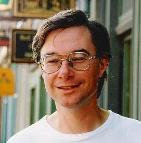Animation of the Figure 8 Knot:We all know what a knot is and what a knot is not. At least we think we do. A knot is what our shoelaces end up in when we try to pull the end of that elegant bow we made out of the lace.

For mathematicians, the definition of a knot has to be more precise. Rather than bothering to define a knot, mathematicians ask the more useful question: when are two knots equivalent topologically? Suppose we are given two simple closed curves in three dimensions. The branch of mathematics called knot theory provides us will tools to check whether one knot can be continuously transformed into another.

A very special case of this problem is to determine whether a knot is equivalent to its mirror image. If we hold some knots up in front of a mirror we can see regard the mirror image as another knot. When it is possible to continuously transform a knot into its mirror image, the knot is said tobe achiral. Otherwise, the knot is said to be chiral. The animation above shows that the figure eight knot seen at the beginning can be transformed into its mirror image, which appears at the end.

A wonderful and accessible book on Knot Theory is Colin C. Adams' The Knot Book, published by Freeman and Co. It requires no advanced mathematics but looks at some deep problems involving knots.